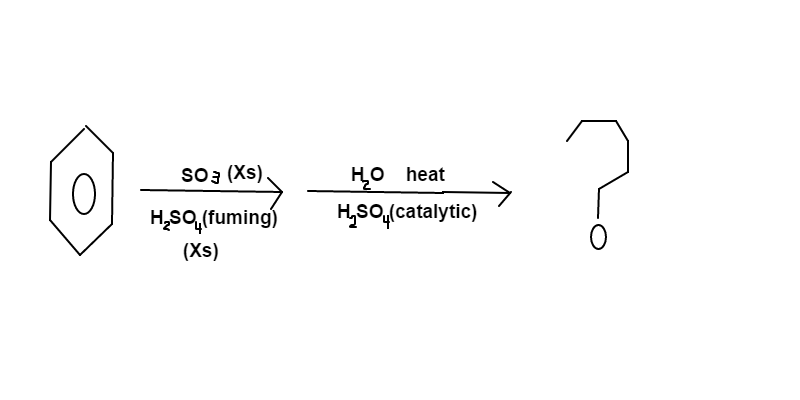# Sulfur Organic Chemistry

Chemistry Level 3Benzene is reacted in a vessel containing an excess (Xs) of $\ce{SO_3}$ and fumming $\ce{H_2SO_4}$. The major product is then transferred to a new vessel containing diluted $\ce{H_2SO_4}$. After heating, the major product in the second vessel will contain $a$ carbon, $b$ hydrogen, $c$ sulfur, and $d$ oxygen atoms.

Find $a+b+c+d$.

David's Organic Chemistry Set

David's Physical Chemistry Set

×